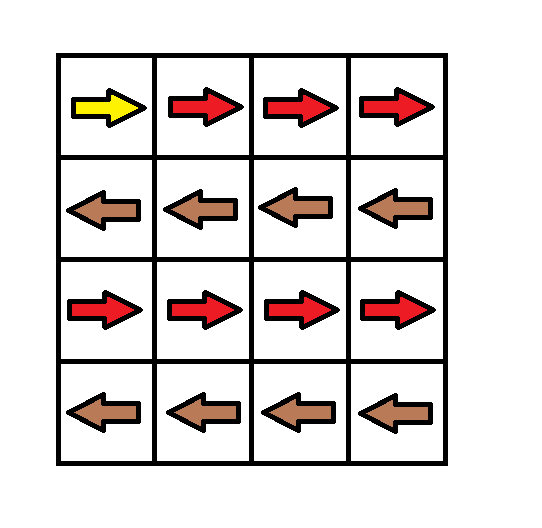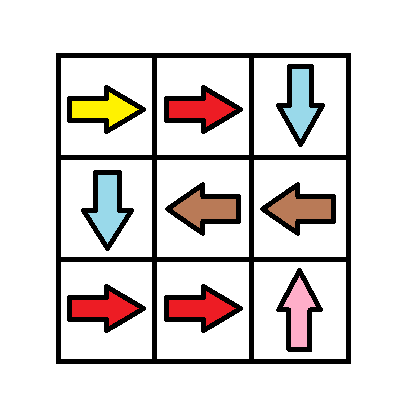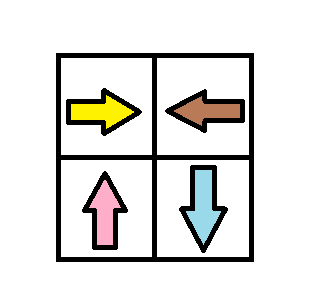1368. Minimum Cost to Make at Least One Valid Path in a Grid

Given a m x n `grid`. Each cell of the `grid` has a sign pointing to the next cell you should visit if you are currently in this cell. The sign of `grid[i][j]` can be:
• 1 which means go to the cell to the right. (i.e go from `grid[i][j]` to `grid[i][j + 1]`)
• 2 which means go to the cell to the left. (i.e go from `grid[i][j]` to `grid[i][j - 1]`)
• 3 which means go to the lower cell. (i.e go from `grid[i][j]` to `grid[i + 1][j]`)
• 4 which means go to the upper cell. (i.e go from `grid[i][j]` to `grid[i - 1][j]`)

Notice that there could be some invalid signs on the cells of the `grid` which points outside the `grid`.

You will initially start at the upper left cell `(0,0)`. A valid path in the grid is a path which starts from the upper left cell `(0,0)` and ends at the bottom-right cell `(m - 1, n - 1)` following the signs on the grid. The valid path doesn't have to be the shortest.

You can modify the sign on a cell with `cost = 1`. You can modify the sign on a cell one time only.

Return the minimum cost to make the grid have at least one valid path.

Example 1:```Input: grid = [[1,1,1,1],[2,2,2,2],[1,1,1,1],[2,2,2,2]]
Output: 3
Explanation: You will start at point (0, 0).
The path to (3, 3) is as follows. (0, 0) --> (0, 1) --> (0, 2) --> (0, 3) change the arrow to down with cost = 1 --> (1, 3) --> (1, 2) --> (1, 1) --> (1, 0) change the arrow to down with cost = 1 --> (2, 0) --> (2, 1) --> (2, 2) --> (2, 3) change the arrow to down with cost = 1 --> (3, 3)
The total cost = 3.
```

Example 2:```Input: grid = [[1,1,3],[3,2,2],[1,1,4]]
Output: 0
Explanation: You can follow the path from (0, 0) to (2, 2).
```

Example 3:```Input: grid = [[1,2],[4,3]]
Output: 1
```

Example 4:

```Input: grid = [[2,2,2],[2,2,2]]
Output: 3
```

Example 5:

```Input: grid = []
Output: 0
```

Constraints:

• `m == grid.length`
• `n == grid[i].length`
• `1 <= m, n <= 100`

1368. Minimum Cost to Make at Least One Valid Path in a Grid
``````struct Solution;

use std::collections::VecDeque;

impl Solution {
fn min_cost(grid: Vec<Vec<i32>>) -> i32 {
let n = grid.len();
let m = grid.len();
let mut dist = vec![vec![std::i32::MAX; m]; n];
let mut queue: VecDeque<(usize, usize, i32)> = VecDeque::new();
dist = 0;
queue.push_back((0, 0, 0));
while let Some((i, j, d)) = queue.pop_front() {
let right = Self::cost(i, j, d, 1, &grid);
let left = Self::cost(i, j, d, 2, &grid);
let down = Self::cost(i, j, d, 3, &grid);
let up = Self::cost(i, j, d, 4, &grid);
if i > 0 && up < dist[i - 1][j] {
dist[i - 1][j] = up;
queue.push_back((i - 1, j, up));
}
if j > 0 && left < dist[i][j - 1] {
dist[i][j - 1] = left;
queue.push_back((i, j - 1, left));
}
if i + 1 < n && down < dist[i + 1][j] {
dist[i + 1][j] = down;
queue.push_back((i + 1, j, down));
}
if j + 1 < m && right < dist[i][j + 1] {
dist[i][j + 1] = right;
queue.push_back((i, j + 1, right));
}
}
dist[n - 1][m - 1]
}

fn cost(i: usize, j: usize, cost: i32, dir: i32, grid: &[Vec<i32>]) -> i32 {
if dir == grid[i][j] {
cost
} else {
cost + 1
}
}
}

#[test]
fn test() {
let grid = vec_vec_i32![[1, 1, 1, 1], [2, 2, 2, 2], [1, 1, 1, 1], [2, 2, 2, 2]];
let res = 3;
assert_eq!(Solution::min_cost(grid), res);
let grid = vec_vec_i32![[1, 1, 3], [3, 2, 2], [1, 1, 4]];
let res = 0;
assert_eq!(Solution::min_cost(grid), res);
let grid = vec_vec_i32![[2, 2, 2], [2, 2, 2]];
let res = 3;
assert_eq!(Solution::min_cost(grid), res);
let grid = vec_vec_i32![];
let res = 0;
assert_eq!(Solution::min_cost(grid), res);
}
``````